## How to Create Increment Number with Texts in Excel

Sometimes we want to fill 1,2,3,4,5… into each cell in a column, for implement this we can enter 1 in the first cell and then drag fill handle down to fill the following cells. But if there are some texts exist with number in cell for example Student001-1-A, we drag down the fill handle, the other cells are only copied the original value, number 001 will not be increased automatically. So, we need to find a simple way that to make number with texts continuous in cells. This article will provide you a simple solution to create increment number with text in cells easily.

Precondition:

See screenshot below. For student, we use 001,002,003…to identify them, we can type text like ‘Student001-1-A’ one by one manually, but if there are 100 or 1000 students, it will spend a long time to finish this task. So we need simple ways to solve this issue.

## 1. Create Increment Number with Texts by ‘&’ in Excel

As we can see the texts are combined as ‘Student00x-1-A’, so we can separate them firstly, then create increment number in one column, and at last combine all texts again. Please see below steps for details.

Step1: Separate texts in column A to two parts.

(Make sure -1-A is saved in Text format, otherwise error will be displayed)

Step2: Select the range covers column C and column D, drag fill handle down to create increment numbers in column C, and text in column D is copied and pasted.

Step3: In cell E2 enter the formula:

`` =C2&D2``

Step4: Drag down the fill handle in column E till reaching the last data.

Step5: Just copy column E, in column A, right click select Paste Special->Paste Values (the first choice).

Then increment numbers within texts are created properly.

## 2. Create Increment Number Within Texts by Formula in Excel

We can see that in texts ‘Student001-1-A’, except ‘001’ part, other parts are unchanged. So we can extract this part, and then create increment number based on it, and then use formula to combine all parts together.

Step1: Separate texts ‘Student001-1-A’ to three parts. In column C save ‘Student’, column D save ‘1’, column E save ‘-1-A’.

Step2: On D2, drag fill handle down to create increment numbers.

Step3: In F2 enter the formula:

`` =\$C\$2&TEXT(D2,"000")&\$E\$2``

Step4: Click Enter to check result.

Step5: Drag the fill handle down to fill cells till the last data.

Then you can refer to above method step#5 to copy and paste data properly.

## 3. Video: Create Increment Number with Texts in Excel

This video will demonstrate how to create an increment number with texts in Excel using the concatenate operator and TEXT formula.

## 4. Related Functions

• Excel Text function
The Excel TEXT function converts a numeric value into text string with a specified format. The TEXT function is a build-in function in Microsoft Excel and it is categorized as a Text Function. The syntax of the TEXT function is as below: = TEXT (value, Format code)…

## How to Convert Numbers to Text

This post explains that how to convert numbers to text format in excel. How to change or convert number to text format with Text function. How to change a number as text format with Format Cells command.  How to convert a number to text using excel concatenate operator.

## 1. Convert Numbers to Text with Text function

If you want to easily convert numbers to text format, you can use the TEXT function to create an excel formula.

Assuming that you want to convert a number value in cell B1 to Text format, you can write down the following formula:

``=TEXT(B1,"0")``

So if you just want the number converted to text without any formatting, you can enter the above formula in the Cell C1, then press Enter key:

You will see that all number are moved to the left side of their cells.

## 2. Convert Numbers to Text with Format Cells Command

You can also use the Format Cells command to convert numbers to text in excel. Just refer to the following steps:

Step1# Select the cells that you want to convert.

Step2# Right click on the selected cells, and click “Format Cells…” from the drop-down list. Then the Format Cells dialog box will appear.

Step3# switch to the Number Tab, then choose Text from the list of formatting categories.

Step4# click OK button.

You will see that the number in selected cells are converted as Text format.

## 3. Convert Numbers to Text with concatenate operator

You can use the concatenate operator to join an empty text string and a number, then it will return a text string just containing the number. So it will be treated as Text string. So you can use the following formula to convert number in Cell B1 to text:

``=""&B1``

Now you can enter the above formula in Cell C1, just see the below screenshot:

## 4. Video: How to Convert Numbers to Text in Excel

In this video, you will learn how to convert numbers to text in Excel using the TEXT function and the Format Cells command.

## 6. Related Functions

• Excel Concat function
The excel CONCAT function combines 2 or more strings or ranges together.This is a new function in Excel 2016 and it replaces the CONCATENATE function.The syntax of the CONCAT function is as below:=CONCAT (text1,[text2],…)…
• Excel Text function
The Excel TEXT function converts a numeric value into text string with a specified format. The TEXT function is a build-in function in Microsoft Excel and it is categorized as a Text Function. The syntax of the TEXT function is as below: = TEXT (value, Format code)…

## How to Convert Military Time to Standard Time in Excel

This post will guide you how to convert military time to standard time with a formula in Excel. How do I convert from military time 17:29:30 to regular time 5:29:30PM in Excel.  How do I Convert Standard time to military time with a formula in Excel.

## 1. Convert Military Time to Standard Time using Formula

Assuming that you have a list of data in range B1:B4, in which contain military time. And you wish to convert those times to standard time. How to do it.

You can a formula based on the TIMEVALUE function, the LEFT function, the MID function and the RIGHT function. The formula is similar to the below:

``=TIMEVALUE(LEFT(B1,2)&":"&MID(B1,3,2)&":"&RIGHT(B1,2))``

Step1: You need to type this formula into a blank cell (at this example, we select Cell C1) and press Enter key, then copy this formula from cell C1 to range C2:C4.

Step2: Then you need to select range C1:C4, and right click on it, select Format Cells from the popup menu list. the Format Cells dialog will open.

Step3: Switch to Number tab in the Format Cells dialog box, and click Time from the Category list box, then select the standard time type in the Type list box. Click Ok button.

Step4: Let’s see the last result:

## 2. Convert Military Time to Standard Time using User Defined Function with VBA Code

If you want to convert Military Time to Standard Time using a User-Defined Function with VBA code, you can follow these steps:

Step1: Press ALT + F11 to open the Visual Basic Editor.

Step2: In the Visual Basic Editor, click on Insert -> Module to insert a new module.

Step3: n the new module, paste the following code. Save the module and return to the Excel workbook.

```Function ConvertMilitaryToStandard_ExcelHow(MilitaryTime As String) As String
Dim Hour As Integer
Dim Minute As String
Dim Second As String
Dim StandardTime As String

Hour = Left(MilitaryTime, 2)
Minute = Mid(MilitaryTime, 3, 2)
Second = Right(MilitaryTime, 2)

If Hour = 0 Then
StandardTime = "12:" & Minute & ":" & Second & " AM"
ElseIf Hour < 12 Then
StandardTime = Hour & ":" & Minute & ":" & Second & " AM"
ElseIf Hour = 12 Then
StandardTime = "12:" & Minute & ":" & Second & " PM"
Else
StandardTime = Hour - 12 & ":" & Minute & ":" & Second & " PM"
End If

ConvertMilitaryToStandard_ExcelHow = StandardTime
End Function```

Step4: In a blank cell, enter the following formula:

``=ConvertMilitaryToStandard_ExcelHow(B1)``

Where B1 is the cell containing the Military Time.

Step5: Press Enter to see the converted time in Standard Time format.

The above VBA code converts the Military Time to Standard Time by extracting the hour and minute components of the Military Time and then using an If-Else statement to determine the corresponding Standard Time.

## 3. Convert Standard Time to Military Time Using Text Formula

If you want to convert Standard time to Military time, you can use another formula based on the TEXT function. Like this:

``=TEXT(C1,"HHMMSS")``

Type this formula into cell D1, press Enter key on your keyboard. Drag the AutoFill Handle over to other cells to apply this formula.

## 4. Video: Convert Military Time to Standard Time in Excel

This video will show you how to convert military time to standard time in Excel using both a formula and VBA code.

## 5. Related Functions

• Excel TIMEVALUE Function
The Excel TIMEVALUE function returns the decimal number of the time represented by a text string. so it will convert a time represented by a text string into an Excel time value.The syntax of the TIMEVALUE function is as below:=TIMEVALUE (time_text)…
• Excel MID function
The Excel MID function returns a substring from a text string at the position that you specify.The syntax of the MID function is as below:= MID (text, start_num, num_chars)…
• Excel LEFT function
The Excel LEFT function returns a substring (a specified number of the characters) from a text string, starting from the leftmost character.The LEFT function is a build-in function in Microsoft Excel and it is categorized as a Text Function.The syntax of the LEFT function is as below:= LEFT(text,[num_chars])…
• Excel RIGHT function
The Excel RIGHT function returns a substring (a specified number of the characters) from a text string, starting from the rightmost character.The syntax of the RIGHT function is as below:= RIGHT (text,[num_chars])…
• Excel Text function
The Excel TEXT function converts a numeric value into text string with a specified format. The TEXT function is a build-in function in Microsoft Excel and it is categorized as a Text Function. The syntax of the TEXT function is as below: = TEXT (value, Format code)…

## How to Convert Julian Date to a Calendar date in Excel

This post will guide you how to convert Julian date to a calendar date in Excel. How do I convert a given Julian Date to a calendar date with a formula or VBA Code in Excel.

## 1. What is Julian Date?

Julian day number is the integer assigned to a whole solar day in the Julian day count starting from noon Universal time, with Julian day number 0 assigned to the day starting at noon on Monday, January 1, 4713 BC, proleptic Julian calendar (November 24, 4714 BC, in the proleptic Gregorian calendar), a date at which three multi-year cycles started (which are: Indiction, Solar, and Lunar cycles) and which preceded any dates in recorded history.

## 2. Convert Julian Date to Calendar date Using Formula

If you want to convert Julian date to a calendar date for your date values, and you can use a formula based on the IF function, the LEFT function and the MOD function. Like this:

``=("1/1/"&(IF(LEFT(B2,2)*1<20,2000,1900)+LEFT(B2,2)))+MOD(B2,1000)-1``

Type this formula into cell c2, and press Enter key on your keyboard to apply this formula.

Then go to HOME tab, select Date as Number Format in the Number group.

Keep to select the Cell C2, and drag the AutoFill Handle down to other cells to apply this formula to convert the Julian date format into Calendar date.

## 3. Convert Julian Date to Calendar Date with VBA Code

You can also use a User Defined function with VBA Code to convert Julian date to a Calendar date in Excel. just do the following steps:

Step1: open the VBA editor in Excel by pressing Alt + F11

Step2:  Insert a new module and paste the below code into the module.

```Function JulianToCalendar_ExcelHow(julianDate As Double) As Date
Dim year As Integer
year = IIf(Left(julianDate, 2) < 20, 2000, 1900) + Left(julianDate, 2)
JulianToCalendar_ExcelHow = DateSerial(year, 1, 1) + (julianDate Mod 1000) - 1
End Function```

Step3: you can use the formula in a blank cell to convert it to a calendar date.

``=JulianToCalendar_ExcelHow(B2)``

This function takes a Julian date as input (in the format of a double) and returns a calendar date (in the format of a Date object).

## 4. Convert calendar date to Julian date using Formula

To convert a calendar date to a Julian date in Excel, you can use the following formula:

``=TEXT(C2,"yy")&TEXT((C2-DATEVALUE("1/1/"&TEXT(C2,"yy"))+1),"000")``

where C2 is the cell containing the calendar date you want to convert.

Let’s See How this Formula Works:

• The TEXT function is used to convert the year portion of the date to a two-digit number in YY format. The formula TEXT(C2,”yy”) extracts the last two digits of the year from the date in cell C2.
• The DATEVALUE function is used to convert a text string in the format “1/1/YY” to a date value. The formula DATEVALUE(“1/1/”&TEXT(C2,”yy”)) returns the date value for January 1 of the same year as the date in cell C2.
• Subtracting the January 1 date value from the date value in cell C2 gives the number of days between January 1 and the date in cell C2.
• Adding 1 to this number gives the day of the year for the date in cell C2.
• The TEXT function is used again to format the day of the year as a three-digit number with leading zeros. The formula TEXT((C2-DATEVALUE(“1/1/”&TEXT(C2,”yy”))+1),”000″) returns the day of the year in DDD format.

## 5. Convert calendar date to Julian date with VBA Code

You can create a User Defined function with VBA Code to convert a calendar date to a Julian date in Excel, just do the following steps:

Step1: open the VBA editor in Excel by pressing Alt + F11

Step2:  Insert a new module and paste the below code into the module.

```Function ConvertToJulianDate_ExcelHow(myDate As Date) As String
Dim myYear As String
Dim julianDay As String

myYear = Format(myDate, "yy")
julianDay = Format(myDate - DateSerial(year(myDate), 1, 1) + 1, "000")

ConvertToJulianDate_ExcelHow = myYear & julianDay
End Function
```

Step3: You can then call the function from a worksheet cell or from another VBA macro.

For example, to convert the date in cell C2 to a Julian date in cell E2, you could use the formula in cell E2.

``=ConvertToJulianDate_ExcelHow(C2)``

The function calculates the Julian date by extracting the year from the calendar date using the Format function, and then subtracting the date of January 1 of that year from the calendar date, adding 1 to get the number of days since January 1, and then formatting the result as a 3-digit number using the Format function.

## 6. Video: Convert between Julian date and Calendar Date in Excel

In this video, you will learn how to convert between Julian dates and calendar dates in Excel using both formulas and VBA code.

## 7. Related Functions

• Excel IF function
The Excel IF function perform a logical test to return one value if the condition is TRUE and return another value if the condition is FALSE. The IF function is a build-in function in Microsoft Excel and it is categorized as a Logical Function.The syntax of the IF function is as below:= IF (condition, [true_value], [false_value])….
• Excel MOD function
he Excel MOD function returns the remainder of two numbers after division. So you can use the MOD function to get the remainder after a number is divided by a divisor in Excel. The syntax of the MOD function is as below:=MOD (number, divisor)….
• Excel LEFT function
The Excel LEFT function returns a substring (a specified number of the characters) from a text string, starting from the leftmost character.The LEFT function is a build-in function in Microsoft Excel and it is categorized as a Text Function.The syntax of the LEFT function is as below:= LEFT(text,[num_chars])…t)…

## How to Generate Random Time in Excel

In some special situations we may need to generate some random times in worksheet. We can enter time manually and one by one, but if we want to require a lot of random dates in excel worksheet, we will spend a lot of time on manual entry, and this work is very boring.

We need to find out a quick and convenient way to insert random times in excel, this tutorial will help you to solve this problem by enter formula with TEXT and RAND functions and VBA Code.

## 1. Generate Random Time in Excel

Step1: Select one cell, enter the formula:

`` =TEXT(RAND(),"HH:MM:SS")``

The RAND function returns a random number, TEXT function can convert number to a proper format, in this formula we convert number to HH:MM:SS format.

Step2: Click Enter to get the result. Verify that a random time is displayed.

Step3: We just need to drag fill handle down to create more random times. You can also copy this formula to other cells to get more random times.

Comment:

Actually, you can also only use RAND function itself to get a random time here. For example, enter =RAND() in cell A1. Then we get a real number.

In Home ribbon, click General dropdown list in Number group.

Select Time format.

Then we will get a random time properly.

## 2. Generate Random Time Between Two Times in Excel

Step1: For RAND function, if you want to generate a number between a and b, we can use =RAND()*(b-a)+a to get a random number. So if we want to get a random time between a and b, we can use formula =TEXT(RAND()*(b-a)/24+a/24,”HH:MM:SS”). So, if we want get a random time between 7 to 13 o’clock, in cell A1, enter：

``=TEXT(RAND()*(13-7)/24+7/24,"HH:MM:SS")``

In above screenshot, we can see that returned random time 10:33:13 can meet our expectation. As we mentioned above, we can also only use RAND function here as well, enter =RAND()*(13-7)/24+7/24, then convert it to time format.

Step2: Drag fill handle down. Verify that random times between time 7 and 13 o’clock are displayed properly.

## 3. Generate Random Date and Time in Excel

Step1: Refer to above parts, we can update the formula to get date and time properly. Enter the formula

``=TEXT(RAND()*("1/1/2019 7:00:00" - "12/31/2019 13:00:00")+"12/31/2019 13:00:00","MM/DD/YYYY HH:MM:SS")``

Verify that a random date and time is returned properly. You can change start and end date time to meet your demands.

## 4. Generate Random Time with VBA Code

To generate random time values in Excel using VBA code, you can use the following steps:

Step1: Press the ‘Alt + F11’ keys to open the VBA Editor.

Step2: Click on ‘Insert’ in the VBA menu and select ‘Module’.

Step3: In the new module window, enter the following VBA code:

```Function RandomTime_excelhow() As Date
Randomize
RandomTime_excelhow = TimeSerial(Int(Rnd() * 24), Int(Rnd() * 60), Int(Rnd() * 60))
End Function```

Step4: Save the module and return to the Excel workbook. In any blank cell, enter the following formula to generate a random time value.

``=RandomTime_excelhow()``

You can also use the VBA code to generate random time values in a range of cells by using a loop to iterate over the cells and calling the RandomTime function for each cell.

```Sub GenerateRandomTime()
Dim rng As Range
Set rng = Application.InputBox("Select the range you want to generate random time values", Type:=8)

Dim startTime As Date
startTime = TimeSerial(9, 0, 0) 'Start time is 9:00 AM

Dim endTime As Date
endTime = TimeSerial(17, 0, 0) 'End time is 5:00 PM

Dim cell As Range
For Each cell In rng
cell.Value = startTime + TimeSerial(Int(Rnd() * (endTime - startTime)), Int(Rnd() * 60), Int(Rnd() * 60))
Next cell
End Sub```

## 5. Video: Generate Random Time in Excel

In this video, I will show you how to generate random time values in Excel using both formulas and VBA code.

## 6. Related Functions

• Excel Text function
The Excel TEXT function converts a numeric value into text string with a specified format. The TEXT function is a build-in function in Microsoft Excel and it is categorized as a Text Function. The syntax of the TEXT function is as below: = TEXT (value, Format code)…
• Excel RAND function
The Excel RAND function returns a random real number that is greater than or equal to 0 and less than 1. The syntax of the RAND function is as below:=RAND ()…

## How to Concatenate Cells and keeping Date Format in Excel

This post will guide you on how to combine text and date into the same cell in Excel while keeping the date format in Excel 2010/2013/2016 or Excel 365. We will show you how to concatenate text and date while preserving the date format, using both formula and VBA code methods.

In some cases, you may want to merge text with a date to create a more informative label or heading for your worksheet. However, when you concatenate cells that contain dates, the resulting string may not retain the original date format. This can be problematic when you need to sort or filter data by date.

## 1. Video: Concatenate Cells and keeping Date Format

If you want to learn how to concatenate cells and keep the date format in Excel, you can explore both the formula and VBA code methods in this video.

## 2. Concatenate Cells and Keeping Date Format using Formula

Assuming that you have a list of data in range A1:B4, which contain product names and dates. And you want to concatenate product name and date value into one cell and keeping a certain date format. How to do it. You can use a formula based on the CONCATENATE function and the TEXT function to achieve the result.  Like this:

``=CONCATENATE(A1," ",TEXT(B1,"m/dd/yyyy"))``

Type this formula into a blank cell（D1) and press Enter key. And then drag the AutoFill handle over to other cells to apply this formula.

Note:

The “Format Code” can be used in the excel Text function are shown in the below table.

## 3. Concatenate Cells and Keeping Date Format with VBA Code

You can also use an VBA code to concatenate the selected cells and keeping all date as the original format in Excel. You just need to do the following steps:

Step1: Open your worksheet where you want to concatenate the text and date.

Step2: Press Alt + F11 to open the Visual Basic Editor.

Step3: In the Visual Basic Editor, go to Insert > Module.

Step4: In the module window, paste the following VBA code.

```Sub ConcatenateDateAndText_excelhow()
Dim rng As Range
Dim destCell As Range
Dim rowRange As Range
Dim cell As Range

On Error Resume Next
Set rng = Application.InputBox("Select the range of cells to concatenate", "Select Range", Type:=8)
Set destCell = Application.InputBox("Select the destination cell", "Select Destination", Type:=8)
On Error GoTo 0

If rng Is Nothing Then
MsgBox "No range selected"
Exit Sub
End If

If destCell Is Nothing Then
MsgBox "No destination cell selected"
Exit Sub
End If

' Loop through each row in the selected range
For Each rowRange In rng.Rows
' Concatenate the values in each row while preserving the date format
destCell.Value = rowRange.Cells(1, 1).Value & " " & Format(rowRange.Cells(1, 2).Value, "dd/mm/yyyy")
Set destCell = destCell.Offset(1, 0)
Next rowRange
End Sub```

Step5: Press Alt + F8 to open the Macro dialog box. And select the ConcatenateDateAndText_excelhow macro and click the Run button.

Step6: select the range of cells that you want to concatenate.

Step7: select a destination cell for the concatenated text and date. click OK.

Step8: The macro will run and concatenate the text and date in each row of the selected range while preserving the date format. The concatenated values will be written to the column starting from the selected destination cell.

## 4. Conclusion

Concatenating cells while preserving the date format can be easily achieved in Excel using either a formula or a VBA code.

The formula method involves using the TEXT function to convert the date value into a text string with a specific date format, then concatenating it with the other text values.

The VBA method involves using a macro that loops through each row of a selected range of cells, concatenates the text and date values in each row while preserving the date format.

## 5. Related Functions

• Excel Text function
The Excel TEXT function converts a numeric value into text string with a specified format. The TEXT function is a build-in function in Microsoft Excel and it is categorized as a Text Function. The syntax of the TEXT function is as below: = TEXT (value, Format code)…

## Phone Number Format in Excel

Sometimes when we import phone numbers from external sources, the formatting of the phone numbers is removed. Even if in some situations the phone numbers are not imported from external resource, sometimes the phone numbers in worksheet are not displayed in the correct format, which is very common in daily work. When we are faced with a string of unformatted phone numbers, we find it very confusing and difficult to see the layout of the phone number. Therefore, it is very important for us to learn how to format phone numbers in Excel worksheets and Google worksheets.

In today’s tutorial, we will introduce how to format phone numbers through Excel built-in cell format function, Excel function or formula. These functions can be applied directly to Microsoft Excel worksheet and Google sheet to format phone numbers. We can also customize the formatting in excel worksheets and google worksheets to format different phone number formats depend on different countries.

In addition to how to format phone numbers, we also introduce the phone number formats of some countries, including USA, UK, France, China, Brazil, etc. Each country offers two phone number formats depending on the international or domestic call you are dialing from. We also list the ways to format phone numbers for these countries.

## Format Phone Numbers to Built-in Excel Format

Let’s start with the built-in “Format Cells” function in Excel to convert number format to phone number format.

In excel, there is a self-contained phone number format. This feature is available in Excel worksheets, Google worksheets. In worksheet, there is a special format in “Format Cells” options to format phone numbers according to the position of the numbers. The layout of phone numbers may vary from country to country in the number of digits and symbols, such as the placement of parentheses and hyphens. Here are the specific steps to perform this operation.

Below steps are operated on Excel 2016 for Mac.

STEP 1# Select the cell or a list that needs to be formatted.

STEP 2# In the Home tab (Home tab is displayed on the top in the ribbon), select the Format drop-down menu, then select “Format cells…“. You can also just right-click on your selection and select “Format Cells…” from the pop-up menu.

STEP 3# In the pop-up “Format Cells” dialog box, select the fist “Number” tab, click “Special” from the Category list, then you can see a list of formatting types on the right side of the dialog box.

STEP 4# Select the “Phone Number” option from the list. Then you can see that your phone number is formatted correctly in Sample field. In our example, 2134567890 is formatted to (213)456-7890.

STEP 5# Click “OK” to apply this format to all phone numbers in the selected range.

It should be noted that the format of the cell can not be text, this function does not work well for text. If there is a leading “0”, we need to remove this “0” first, and then use custom formatting to add “0” back. Let’s look at the following example of custom formatting.

## Format Phone Numbers to A Custom Format

If you do not find a preset format that meets your requirements in Excel worksheet or Google worksheet, you can set up a custom format via “Format Cell” function to display the numbers as phone numbers. Again, we still use the “Cell Format” setting to apply custom format to the numbers. Here are the steps for formatting a phone number using custom format.

STEP 1# Select the cell or a list that needs to be formatted.

STEP 2# In the Home tab (Home tab is displayed on the top in the ribbon), select the Format drop-down menu, then select “Format cells…“. You can also just right-click on your selection and select “Format Cells…” from the pop-up menu.

STEP 3# In the pop-up “Format Cells” dialog box, select the fist “Number” tab, click “Custom” from the Category list, then you can see a list of formatting types on the right side of the dialog box. Some types are preset; some are saved in daily work per your demand.

STEP 4# You can select a built-in format that is most similar to the one you want to create. If there is not a similar one, you can type a proper phone number format you want in “Type” textbox. Then custom phone number format is reflected in Sample field. Note that all symbols entered, including spaces, will be reflected in the phone number format.

Here we enter (###) ###-#### to reflect phone number. Each # represents a number.

STEP 5# Click “OK” to apply this format to all phone numbers in the selected range.

Custom format helps us format phone numbers without restrictions. Using a good concatenation symbol, we can enter any phone number format we want. We can also enter zero and plus sign directly into the custome phone number format.

## Phone Numbers with Preceding “+” Sign or Leading “0”

Enter (0###) ###-#### in Type:

Enter 0 (###) ###-#### in Type:

You can add +1 in front of all phone numbers:

Enter (+1) (###) ###-#### in Type:

Using the types listed above we can add a leading 0 or country code (or area code) to a phone number if the original phone number does not have a leading 0 or code.

## Format Phone Number with Excel TEXT Function

The text function can quickly handle phone numbers with the same format.

TEXT function syntax:

`=TEXT(value, format_text)`

### Format a phone number

In the cell just next to phone number, enter =TEXT(A1,”(###) ###-####”).

### Format a phone number table

In my example, the phone numbers are saved in a table, so when you enter the TEXT function in B2, the value parameter is the phone numbers table, and when you press ENTER to run TEXT, a new table is created.

### Format phone numbers with different formats (different lengths)

If phone numbers of different lengths are mixed together, the TEXT function alone cannot help us format the phone numbers, so we need the help of the IF function. We can first sort the phone numbers by region or length of the phone number, and then use the IF and TEXT functions to format the phone numbers. Note that this formula can only format two types of phone numbers.

For example, the length of the phone number is different. Some contain 11 digits and some contain 10 digits. For phone number with 11 digits, format text to “(###) # ###-####”, for phone number with 10 digits, format text to “####-###-###”.

`=TEXT([@[Phone Number]],IF(LEN([@[Phone Number]])<11,"####-###-###","(###) # ###-####"))`

## List of International Phone Number Format Standards

International standards for phone number formats are issued by the International Telecommunication Union. Each country and region has its own way of writing phone numbers. Even for the same phone number, the format of the phone number is different for domestic calls and international calls.

A standard phone number should be “+ (country code) (area code) phone number”. For example, +(1)(425) 555-0100 is the phone number for the U.S. If you are calling a U.S. phone number, their phone number starts with +1, followed by the correct 10 digits of the phone number (the first three are the area code). The other countries follow the similar rules.

When dialing an international call, you need to add 001 (the U.S. international call area code) plus the U.S. local area code in front of phone number or cell phone number.

For example, in the dialing screen, type 001 860 xxxx xxxx, you can call Connecticut from abroad. Here 00 is the international call access code, the leading 0 is usually ignored in writing.

US Phone Number Format

International Call +1 860 XXX XXXX

Domestic Call +0860 XXX XXXX

(The prefix code 860 is the area code for Connecticut, USA)

UK Phone Number Format

International Call +44 20 XXXX XXXX

Domestic Call +020 XXXX XXXX

(The prefix code 20 is the area code for London, UK)

Australia Phone Number Format

International Call +61 2 XXXX XXXX

Domestic Call +02 XXXX XXXX

(The prefix code 2 is the area code for Sydney, Australia)

People’s Republic of China Phone Number Format

International Call +86 10 XXXX XXXX

Domestic Call +010 XXXX XXXX

(The prefix code 10 is the area code for Beijing, RPC)

Japan Phone Number Format

International Call +81 3 XXXX XXXX

Domestic Call +03 XXXX XXXX

(The prefix code 3 is the area code for Tokyo, Japan)

South Korea Phone Number Format

International Call +82 2 XXX XXXX

Domestic Call +02 XXX XXXX

(The prefix code 2 is the area code for Seoul, South Korea)

India Phone Number Format

International Call +91 80 XXXX XXXX

Domestic Call +080 XXXX XXXX

(The prefix code 80 is the area code for Bangalore, India)

### Related Functions

• Excel IF function
The Excel IF function perform a logical test to return one value if the condition is TRUE and return another value if the condition is FALSE. The IF function is a build-in function in Microsoft Excel and it is categorized as a Logical Function.The syntax of the IF function is as below:= IF (condition, [true_value], [false_value])….
• Excel Text function
The Excel TEXT function converts a numeric value into text string with a specified format. The TEXT function is a build-in function in Microsoft Excel and it is categorized as a Text Function. The syntax of the TEXT function is as below: = TEXT (value, Format code)…

To pad a number with zeros, you need to add leading spaces and/or carriers. For example, if the original digit contains 2 digits, three additional characters will be added on before it. If there are 3 digits in total, allocating two places for these extra pieces of information ensures that your output looks correct without having any factual inaccuracies within them!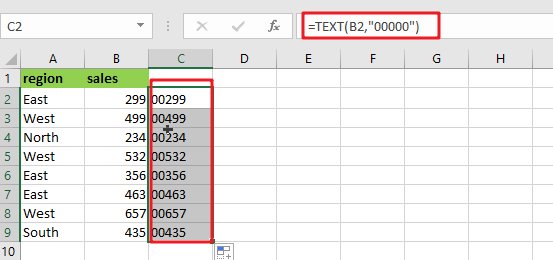Two ways to solve this problem: you can either convert the number into text with leading zeros or apply a custom format that displays numbers As desired. The best approach depends on your application’s needs and how much flexibility there will be in future renovations to accommodate new needs. If converting all numbers back from their textual representation would entail too much work for someone else who might come along later down the line, then it may actually make more sense optically employing an option where some values remain numeric-although not necessarily negative, though!

## Method1：Convert To Text Using TEXT Function

Leading zeros can be added easily by using the TEXT function. For example, if you want five characters total and use “00000” as your number format in Microsoft Excel, it will return those values after conversion from numbers into text with that specific style!

`=TEXT(B2,"00000")   `With the text “00299“,  Excel automatically aligns it to the left, but you can set alignment manually as you want. To replace original numbers with converted values: copy them first and then select all occurrences of this string in your workbook before pressing Paste Special > Values which will convert those contents into an entry on its own without changing anything else around it, including spacing or font size – very useful if there are many instances!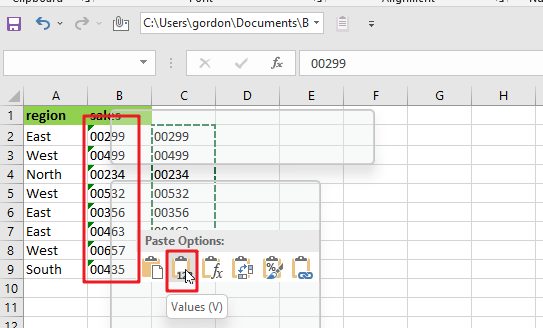## Method2: Apply Number Format

You can also add leading zeros to numbers by applying a custom number format in Excel. However, the key thing you need to understand with this option is that it only changes how your numbers are displayed. Not their underlying value or anything else about them like the size, for instance!

Displaying leading zeros is a simple task with Excel’s Number format command. Select values in B2:B9 and use Control + 1 on your keyboard to open up the Format Cells window to apply this formatting.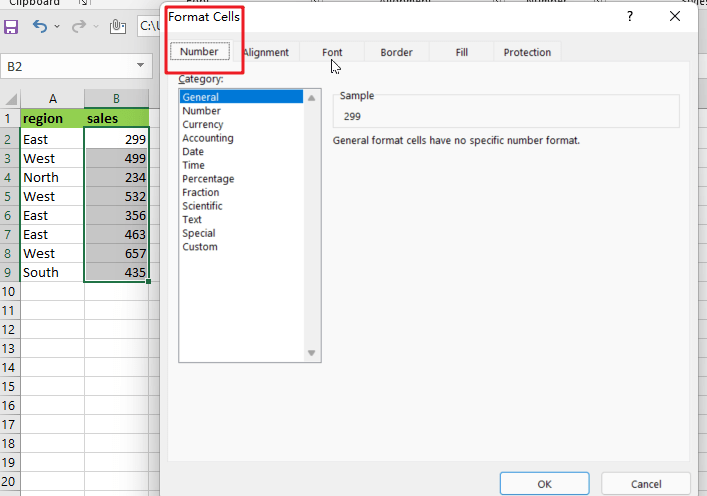Go to the Number tab, Choose “Custom,” and in the input area, “Type” enter 00000.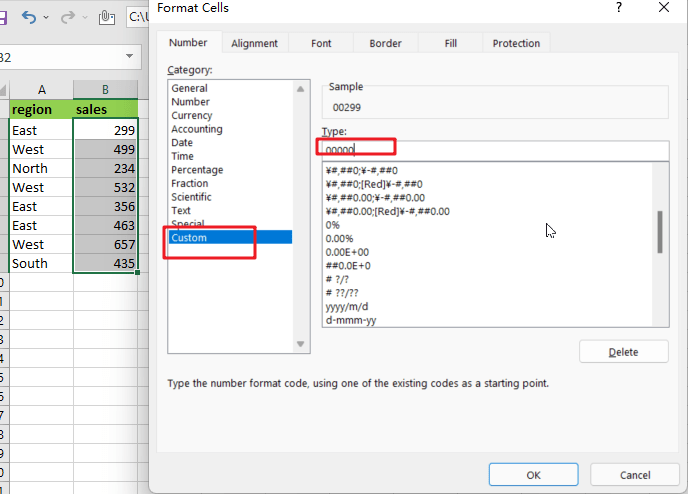The format is applied to the first cell in your selection. Then, you can either press Enter or click “OK” once you’ve made a valid choice, and numbers are displayed with leading zeros as well! If there was no number present before this process began, then one will be created for every cell that has an input value of at least 1.”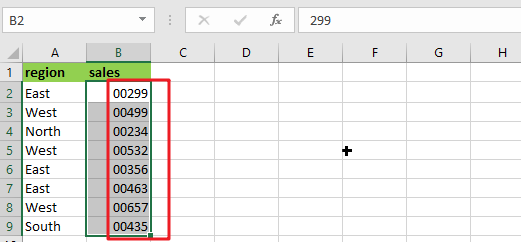### Related Functions

• Excel Text function
The Excel TEXT function converts a numeric value into text string with a specified format. The TEXT function is a build-in function in Microsoft Excel and it is categorized as a Text Function. The syntax of the TEXT function is as below: = TEXT (value, Format code)…

## How to Auto Fill Weekdays or Weekends in Excel

Sometimes we may want to enter a sequence of days in excel for some purpose, and we can press Ctrl+; to insert current date into cell, and then drag the cell down to attach following days into other cells, then we can get a list of days.

But for some special requirement, we just want to fill weekdays or weekends into a list, for example if weekdays exist in the list, weekends are excluded. So, we need to find a way to only insert weekdays or weekends simply. In this article, we will show you the ways to fill days into list per your requirement properly, we provide two ways to fill weekdays or weekends, by Auto Fill function or the formula.

## Method 1: Auto Fill Weekdays in Excel

Step 1: Launch excel open a new worksheet, enter the start date into any cell, if you want to fill only weekdays into the list, please enter a weekday, then drag it down to the next cells till we get the end date in date list. For example, we want to fill weekdays from 9/1/2020 to 9/15/2020, then enter 9/1/2020 in any cell, then drag it down to auto fill days till we get 9/15/2020.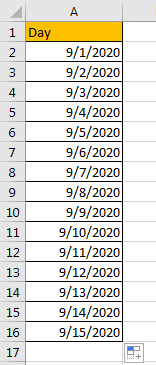Step 2: You may notice that when we dragging the cell down to apply formula to other cells, Auto Fill Options icon is displayed and attached to the last cell. Click on Auto Fill Options icon to load all options. Verify that ‘Fill Weekdays’ option is included.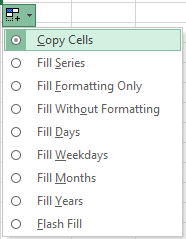Step 3: Check on ‘Fill Weekdays’ option, verify that days are updated from previous 9/1/2020 to 9/21/2020 automatically, that’s because weekends are removed from the list and weekdays are filled instead.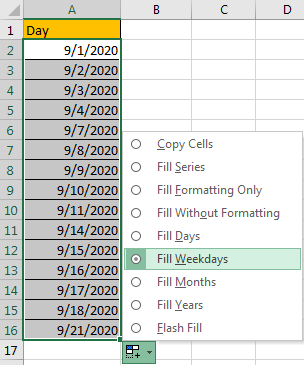Step 4: If you want to keep the end date as 9/15/2020 in your list, just remove the extra days from the list. Now date list with only weekdays is created properly.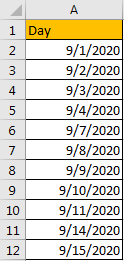## Method 2: Auto Fill Weekends by Formula in Excel

You can see that there is no fill weekends option in Auto Fill Options list. So, we need to find another way to fill weekends only. Actually, you can through below formula to fill weekends properly.

Step 1: Launch excel open a new worksheet, enter the formula =TEXT(“09/05/2020″+INT(ROW(A1)/2)+(CEILING(ROW(A1)/2,1)-1)*6,”mm/dd/yyyy”) into any cell. As you want to fill only weekends into the list, please enter a weekend date into the formula, in this sample we enter 09/05/2020 as start date, you can also change date format ‘mm/dd/yyyy’ to another format.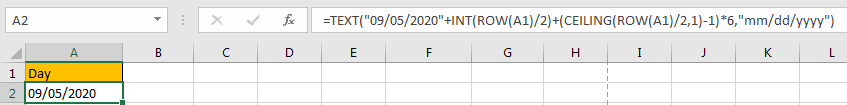Step 2: Then drag it down to the next cells to apply the formula. Till we get the end date in date list. For example, we want to fill all weekends in September, drag it down till weekend date from October occurs.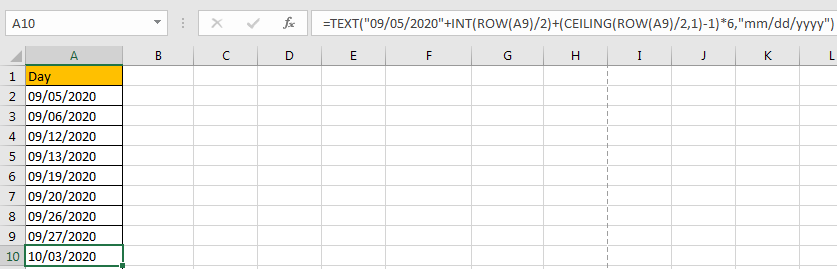Step 3: Just remove the date from October, then weekends from September are listed.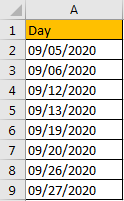### Related Functions

• Excel ROW function
The Excel ROW function returns the row number of a cell reference.The ROW function is a build-in function in Microsoft Excel and it is categorized as a Lookup and Reference Function.The syntax of the ROW function is as below:= ROW ([reference])….
• Excel INT function
The Excel INT function returns the integer portion of a given number. And it will rounds a given number down to the nearest integer.The syntax of the INT function is as below:= INT (number)…
• Excel Text function
The Excel TEXT function converts a numeric value into text string with a specified format. The TEXT function is a build-in function in Microsoft Excel and it is categorized as a Text Function. The syntax of the TEXT function is as below: = TEXT (value, Format code)…
• Excel Ceiling function
The Excel CEILING function returns a given number rounded up to the nearest multiple of a given number of significance.The syntax of the CEILING function is as below:= CEILING (number, significance)

## How to Change Date to The Day of Week in Excel

In excel, we can type a date properly if set cell format as ‘Date’. If there is a list includes multiple dates, is there any way to convert these dates to the days of week accordingly? Actually, there are some tricks to convert date to day of week simply, in this free tutorial we will provide you some little tricks to convert date, you can select one of them to help you to solve your problem.

Precondition:

There are some dates list in ‘Date’ column. Now let’s convert them to day of week and list the result in ‘Day of Week’ column.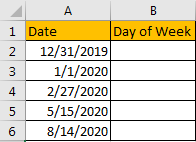## Method 1: Convert Date to Day of Week by Formula

Step 1: In B2 enter the formula =TEXT (A1,”dddd”). TEXT function can change selected value to your expected format.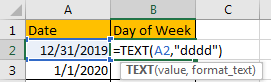Step 2: Click Enter to get result. Verify that Tuesday is returned. In this step, if you apply the formula to convert date format for the first time, a warning message will pop up, just confirm and close it.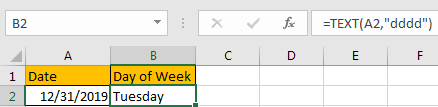Step 3: Drag the fill handle down till reaching the end of the list. Verify that day of week is displayed properly for each date.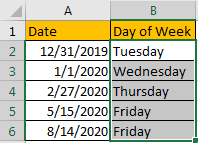## Method 2: Convert Date to Day of Week by Format Cells Feature

Refer to method1 we can see that through changing the format of cells, we can change date to day of week properly. So, we can also change cell format for selected values through excel Format Cells feature.

Step 1: Copy dates in A column to B column firstly. Then select B2:B6, right click to load options, select Format Cells in the last section.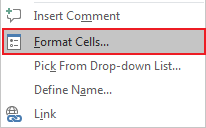Step 2: On Format Cells panel, under Number tab, select Custom in Category list, in Type textbox type ‘dddd’, after typing you can see in Sample section ‘Tuesday’ is displayed.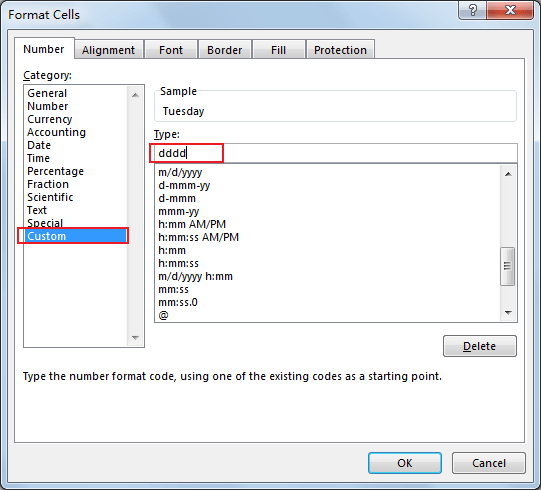Step 3: Click OK and quit Format Cells. Verify that day of week is displayed in each cell properly.## How to Combine Text and Date into one Cell in Excel

This post will guide you how to combine text and dates in the same cell in Excel. How do I combine text and date values into one Cell in Excel 2013/2016.

## Combine Text and Date into One Cell

Assuming that you have a list of data in range B1:C4, and one column contain product names and another column contain date values, and you want to combine both text and date data into one cell. If you just use concatenate operator to combine those two cells, the date value will be displayed as serial number. This is not your expected. See below screenshot: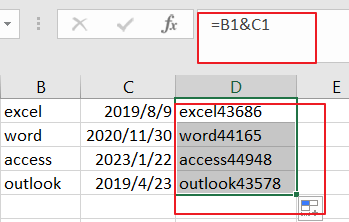To combine Text and date into one cell, you need the TEXT function to change the date format from series number to a text. So you can use the following formula based on the TEXT function:

=B1 & “ “ TEXT(C1,”MM/DD/YYYY”)

Type this formula into a blank cell such as: Cell D1, and drag the AutoFill Handle down to other cells.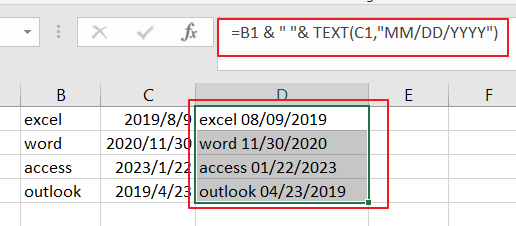### Related Functions

• Excel Text function
The Excel TEXT function converts a numeric value into text string with a specified format. The TEXT function is a build-in function in Microsoft Excel and it is categorized as a Text Function. The syntax of the TEXT function is as below: = TEXT (value, Format code)…

## How to Highlight Cell or Row If Date Is In Current Day/Week/Month in Excel

This post will guide you how to highlight cell if date is the current day or is in the current week or month in Excel. How do I highlight row if date is in current week or month with conditional formatting in Excel 2013/2016.

## Highlight Cell if Date is in Current Day/Week/Month

To highlight the cell of current day or the date is in the current week or month, you can do the following steps:

#1 select the range of cells that you want to highlight date.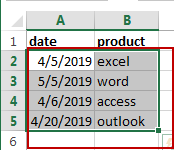#2 go to HOME tab, click Conditional Formatting command under Styles group, and click Highlight Cells Rules menu from the drop down menu list, then select A Date Occurring sub menu. And the A Date Occurring dialog will appear.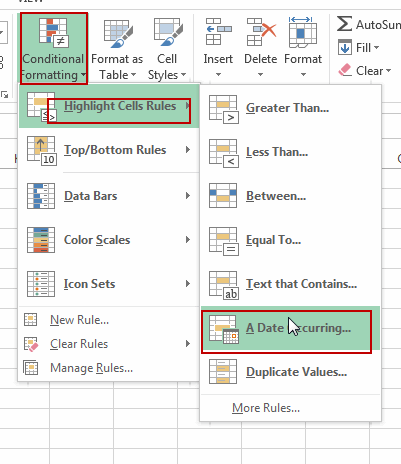#3 if you want to highlight the current day, then select Today option from the first drop down list box in the A Date Occurring dialog box, then choose one color that you want to fill the cell in the second drop down list box. Click Ok button.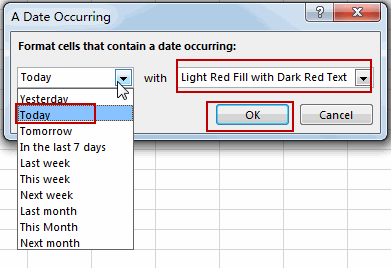Note: if you want to highlight the cell if date is in the current week, just choose This Week option from the first drop down list box. And if you want to highlight the cell if date is in the current month, just choose This Month from the first drop down list box.

#4 you should notice that the current date has been highlighted.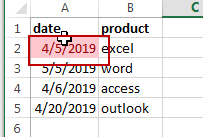## Highlight Row if Date is in Current Day/Week/Month

If you want to highlight the row that If the date in that row is equal to the current day or is in the current week or month, you can do the following steps:

#1 select the entire rows of data that contain date values.#2 go to HOME tab, click Conditional Formatting command under Styles group, Click New Rule from the drop down menu list. And the New Formatting Rule dialog will open.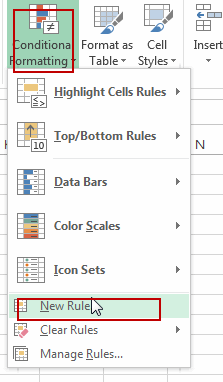#3 click Use a formula to determine which cells to format option in the New Formatting Rule dialog box, type the following formula in the Format values where this formulas is true text box.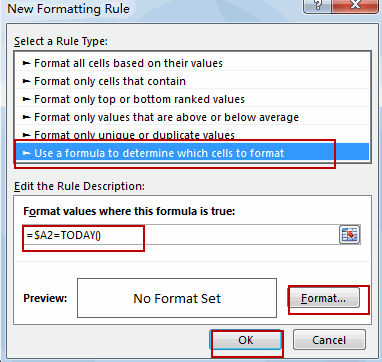To highlight current date, use the following formula:

=\$A2=TODAY()

To highlight row if date is in the current week, use the following formula:

=TODAY()-WEEKDAY(TODAY(), 3)=\$A2-WEEKDAY(\$A2, 3)

To highlight row if date is in the current month, use the following formula:

=TEXT(\$A2,”mmyy”)=TEXT(TODAY(),”mmyy”)

Note: the Cell A2 is the first cell of your selected range.

#4 click Format button, and the Format Cells dialog will open. Click Fill tab, select on color that you want to use. Click Ok button.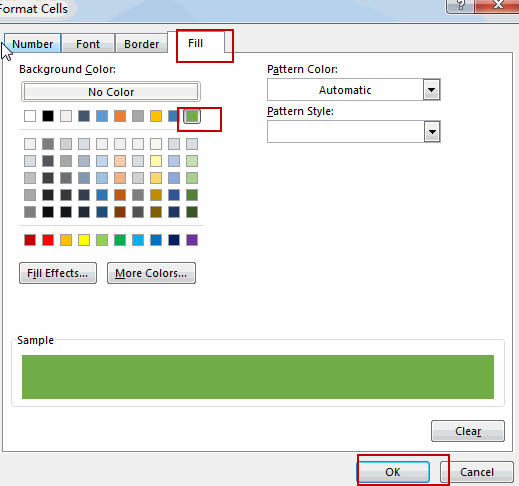#5 click Ok button. You should see that the entire rows which contain the current day have been highlighted.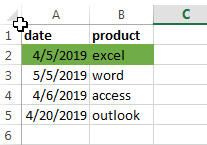### Related Functions

• Excel WEEKDAY function
The Excel WEEKDAY function returns a integer value representing the day fo the week for a given Excel date and the value is range from 1 to 7.The syntax of the WEEKDAY function is as below:=WEEKDAY (serial_number,[return_type])…
• Excel TODAY function
The Excel TODAY function returns the serial number of the current date. So you can get the current system date from the TODAY function. The syntax of the TODAY function is as below:=TODAY()…
• Excel Text function
The Excel TEXT function converts a numeric value into text string with a specified format. The TEXT function is a build-in function in Microsoft Excel and it is categorized as a Text Function. The syntax of the TEXT function is as below: = TEXT (value, Format code)…

## How to Delete or Remove Year from a Date in Excel

This post will guide you how to delete or remove year from a given standard date in Excel. How do I remove the year from a date with a formula in Excel.

## Remove Year from a Date

Assuming that you have a list of data in range B1:B5, in which contain date values. And you want only display the month and Day parts. So you need to remove year from cells. How to do it. You can use a formula based on the MONTH function and the DAY function to achieve the result.  Like this:

`=MONTH(B1)&"-"&DAY(B1)`

Or

`=CONCATENATE(MONTH(B1),"-",DAY(B1))`

Type one of the above formula into cell C1, and press Enter key. And then drag the AutoFill handle from Cell C1 to C5.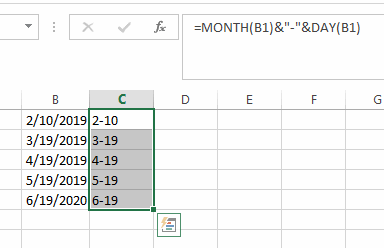You should notice that the year part has been removed for each date in range B1:B5.

You can also use the TEXT function to create a formula to achieve the same result. Like this:

`=TEXT(B1,"mm-dd")`

Type one of the above formula into cell D1, and press Enter key. And then drag the AutoFill handle from Cell D1 to D5.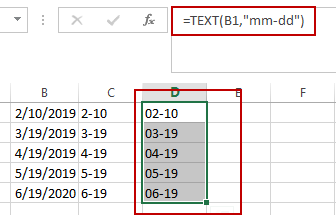### Related Functions

• Excel MONTH function
The Excel MONTH function returns the month of a date represented by a serial number. And the month is an integer number from 1 to 12. The syntax of the MONTH function is as below:=MONTH (serial_number)…
• Excel DAY function
The Excel DAY function returns a day of a date (from 1 to 31).The DAY function is a build-in function in Microsoft Excel and it is categorized as a DATE and TIME Function.The syntax of the DAY function is as below:= DAY (date_value)…
• Excel Text function
The Excel TEXT function converts a numeric value into text string with a specified format. The TEXT function is a build-in function in Microsoft Excel and it is categorized as a Text Function. The syntax of the TEXT function is as below: = TEXT (value, Format code)…

## How to Convert Date to YYYY-MM-DD format in Excel

This post will guide you how to convert the current date to a specified date format in Excel. How do I convert date to YYYY-MM-DD format with Format Cells Feature in Excel. How to convert date format to a specific date format with a formula in Excel.

Assuming that you have a list of data in range A1:A4, in which contain date values with MM/DD/YYYY format. And you need to convert the date to the YYYY-MM-DD format for your selected cells in Excel. How to do it. You can use achieve the result via format cells feature or a formula. Let’s see the following detailed introduction.

## Convert Date to YYYY-MM-DD Format with Format Cell

If you want to convert the selected range of cells to a given YYYY-MM-DD format, you can use the format cells to change the date format. Here are the steps:

#1 select the date values that you want to convert the date format.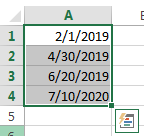#2 right click on it, and select Format Cells from the pop up menu list. And the Format Cells will open.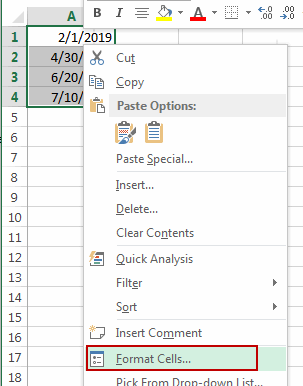#3 switch to the Number tab in the Format Cells dialog box, and click the Custom category under the Category: list box, and enter the format code YYYY-MM-DD into the type text box, and click OK button.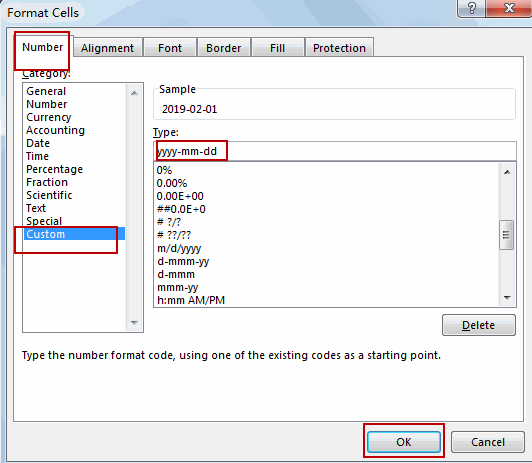#4 the selected date values should be converted to YYYY-MM-DD format.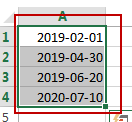## Convert Date to YYYY-MM-DD Format with a Formula

You can also use an Excel formula based on the TEXT function to convert the given date value to a given format (yyyy-mm-dd). Like this:

`=TEXT(A1,"yyyy-mm-dd")`

Type this formula into a blank cell and press Enter key on your keyboard, and then drag the AutoFill Handle over to other cells to apply this formula.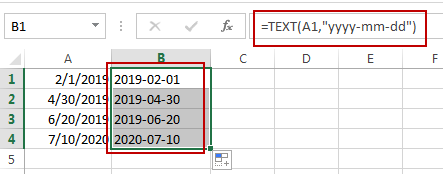## How to Display Negative Time Value in Excel

This post will guide you how to display negative time value in Excel. How do I change the way negative times are displayed in Excel. How to show negative dates or times with a formula in Excel.

If the cell value is a negative time or date value, and the Excel will only show #### error in that cell. So how to correct this error to show the correct negative time or date.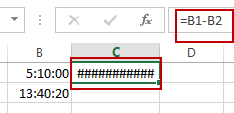## Display Negative Time Value

You can change the Excel’s Default Date System to correct this error. Just do the following steps:

#1 click File tab, and select Options from the menu list. And the Excel Options dialog will open.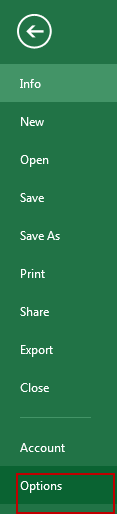#2 click Advanced from the left pane, and check Use 1904 data system under When calculating this workbook section. Click Ok button.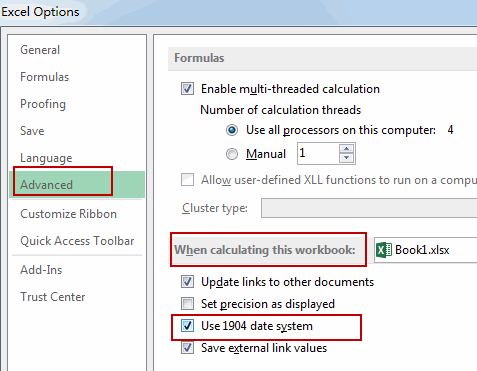#3 the negative time will be shown normally.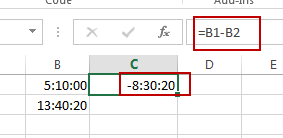## Display Negative Time Value with Formula

You can also use a formula based on the Text function, the Max function and the MIN function to achieve the result of displaying negative time value properly in Excel.

Just like this formula:

`=TEXT(MAX(\$B\$1:\$B\$2)-MIN(\$B\$1:\$B\$2),"-H::MM")`

Type this formula into a blank cell and then press Enter key in your keyboard.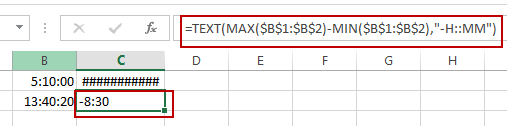You will see that a negative time value will be displayed in that cell.

The combination of the MAX function and the MIN function will subtract the earlier time from the later time, and get the positive time value. And then it will be formatted using the TEXT function so that it shows a negative time.

Note: you should know that the returned result is actually a text value, not a numeric value.

Video: Display Negative Time Value

### Related Functions

• Excel Text function
The Excel TEXT function converts a numeric value into text string with a specified format. The TEXT function is a build-in function in Microsoft Excel and it is categorized as a Text Function. The syntax of the TEXT function is as below: = TEXT (value, Format code)…
• Excel MIN function
The Excel MIN function returns the smallest numeric value from the numbers that you provided. Or returns the smallest value in the array.The MIN function is a build-in function in Microsoft Excel and it is categorized as a Statistical Function.The syntax of the MIN function is as below:= MIN(num1,[num2,…numn])….
• Excel MAX function
The Excel MAX function returns the largest numeric value from the numbers that you provided. Or returns the largest value in the array.= MAX(num1,[num2,…numn])…

## Extract Month and Year from Date in Excel

This post will guide you how to extract month and year from a date in excel. How do I get month and year from date cells  with a formula in Excel. How to convert date to month and year in Excel.

## Extract Month and Year from Date

If you want to extract month and year from a date in Cell, you can use a formula based on the TEXT function.
Assuming that you have a list of data in range B1:B4 that contain dates, and you want to convert the normal excel date into year and month format (yyyymm), How to achieve it. You can use the following format:

`=TEXT(B1,"yyyymm")`

Type this formula into a blank cell and then press Enter key in your keyboard. and drag the AutoFill Handle over other cells to apply this formul.

###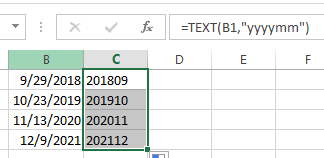This formula will get a date with the year and month only from the orignial date in Cells.

If you only want to extract month from a date, and you can use the following formula:

`=TEXT(B1,"mmm")`

###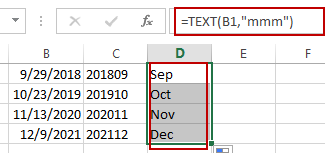If you want to convert date to year format only, you can use the following formula:

`=TEXT(B1,"yyyy")`

### Related Functions

• Excel Text function
The Excel TEXT function converts a numeric value into text string with a specified format. The TEXT function is a build-in function in Microsoft Excel and it is categorized as a Text Function. The syntax of the TEXT function is as below: = TEXT (value, Format code)…

## Convert Date to Text

This post will guide you how to convert dates stored as text format in Excel. How do I convert the date as date format to text format in excel. You can use a variety of formulas to convert the dates in your worksheet to text format.

You can use the TEXT function to create a formula to convert the date as date format to text format.

Assuming that you have a list of data that contain date values and you want to convert its format as text format. Just do the following steps:

#1 type the following formula into a blank cell.

=TEXT(B1,”yyyy-mm-dd”), press Enter key in your keyboard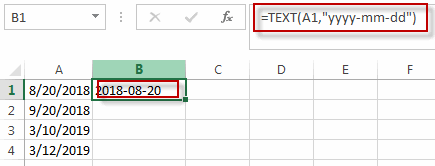#2 drag the AutoFill Handle over other cells to apply this formula.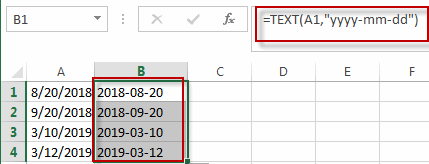If you want to convert a date as Text format to number, you can use the DATEVALUE function or just use the Format Cell function to achieve it. Such as: =DATEVALUE(“2018/23/08”)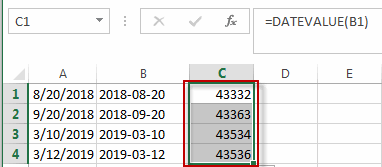### Related Functions

• Excel Text function
The Excel TEXT function converts a numeric value into text string with a specified format. The TEXT function is a build-in function in Microsoft Excel and it is categorized as a Text Function. The syntax of the TEXT function is as below: = TEXT (value, Format code)…
• Excel DATEVALUE Function
The Excel DATEVALUE function returns the serial number of date. And it can be used to convert a date represented as text format into a serial number that recognizes as a date format.The syntax of the DATEVALUE function is as below:=DATEVALUE(date_text)…

## Changing Date Format

This post will guide you how to convert dates between Australian and US American formats in Excel. How to change the data format from your local locale to other locale, such as: US or South Africa. As the different country may be has different data format. So this post will talk that how to change the date Format for different country.

Assuming that you have a list of data that contain dates values and its data format will keep the default format based on your system locale. And you want to change the data format from the current format to Australian data format. How to achieve it. This post will introduce two methods to change the Date Format in your worksheet.

## Changing Date Format with Format Cell Feature

To change the date format as your need in Excel, you can use the Format cell feature, just do the following steps:

#1 select the range of cells that you want to convert date format.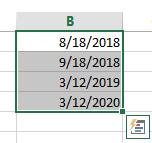#2 right click on the selected range, select Format Cells from the drop-down menu list. And the Format Cells dialog will open.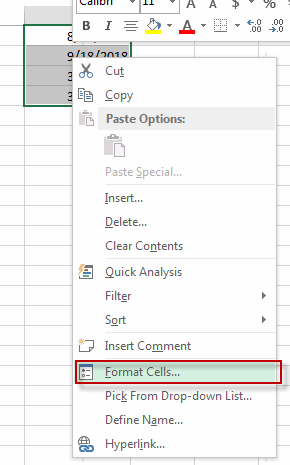#3 click Number tab to switch the Number tab in the Format Cell dialog box, and select Date category from the Category list box. And select the date format from the type list box. And select English (Australia) from the locale drop-down list box. Click Ok button to confirm the date format setting.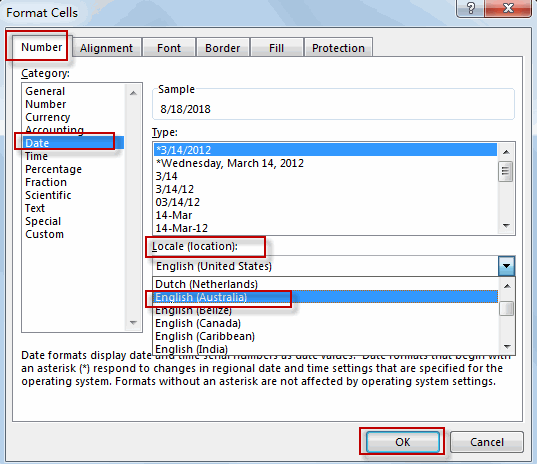#4 the data format in the selected range of cells have been changed as you need.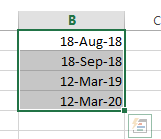## Change Date Format with Formula

You can also use the TEXT function to create a formula to change the date format for the date value in cell in Excel. For example, you want to change the date format to “yyyy/dd/mm”, just use the following formula based on the TEXT function:

`=TEXT(B1,"yyyy/dd/mm")`

Type this formula into a blank cell C1, and press Enter key in your keyboard, and then drag the AutoFill Handle over other cells to apply this formula.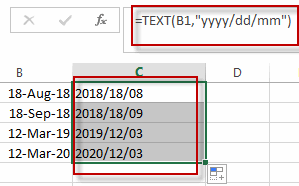You will see that the data format of the date values in the range of B1:B3 haven been changed.

### Related Functions

• Excel Text function
The Excel TEXT function converts a numeric value into text string with a specified format. The TEXT function is a build-in function in Microsoft Excel and it is categorized as a Text Function. The syntax of the TEXT function is as below: = TEXT (value, Format code)…

## Combining Date and Time into One Cell

This post will guide you how to combine a data column and time column into one column in Excel. How do I quickly combine data and time in the different cells into one cell in your Worksheet. For example, if you have two columns and one column contain date values and another column contain time values, and you want to combine date cell and time cell into one single cell, how to achieve it. Let’s see the following detailed steps.

## Combining Date and Time into One Cell

To combine the date in one cell and time in another cell in Excel, you can use the TEXT function in combination with the concatenation operator to create a new formula. Like this:

`=TEXT(B1,"m/dd/yy ")&TEXT(C1,"hh:mm:ss")`

You need to type this formula into a blank cell and then press Enter key in your keyboard, and drag the AutoFill Handle over other cells to apply this formula to combine date and time.

The First Text function will format the text string based on the date format provided in the second argument. And the second Text function will format the text string based on the time format provided in the second argument.

Let’s see the result: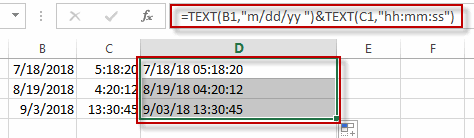You can also directly use add operation to combine date and time values from the different cells into one single cell. Like this:

`=B1 + C1`

Type this formula into a blank cell, and then press Enter key.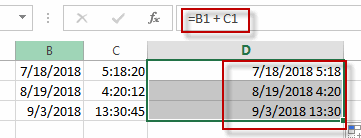### Related Functions

• Excel Text function
The Excel TEXT function converts a numeric value into text string with a specified format. The TEXT function is a build-in function in Microsoft Excel and it is categorized as a Text Function. The syntax of the TEXT function is as below: = TEXT (value, Format code)…

## Convert Month Name to Number

This post will guide you how to convert month name to number in excel. How do I convert month name to number with an excel formula. How to convert month name to number with an Excel VBA macro. How to convert 3 letter month abbreviation to number in excel. How to convert 3 character month to number in excel.

Assuming that you have a list of data B1:B12 contain month names and you want to convert all month names to numbers, how to achieve it.

## Convert Month Name to Number with Excel Formula

If you want to convert month name to number with an excel formula, or you want to convert 3 letter month name to numbers, you can create a formual based on the MONTH function and the DATEVALUE function. Like this:

`=MONTH(DATEVALUE(B1&"1"))`

Type this formula into the formula box of a blank cell C1, then drag the AutoFill handler over other cells to apply this formula.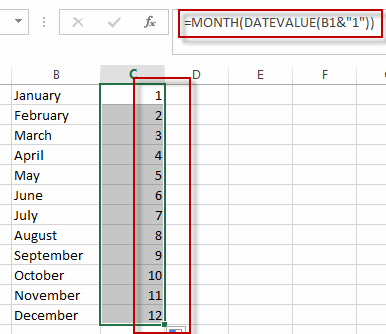You will see that all month names have been converted to numbers in range of cells C1:C12.

## Convert Month Name to Number with VBA code

You can also use an excel VBA Macro to convert month name to number in excel. Just do the following steps:

#1 open your excel workbook and then click on “Visual Basic” command under DEVELOPER Tab, or just press “ALT+F11” shortcut.#2 then the “Visual Basic Editor” window will appear.

#3 click “Insert” ->”Module” to create a new module#4 paste the below VBA code into the code window. Then clicking “Save” button.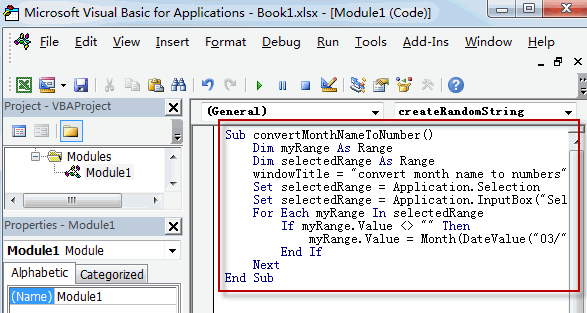```Sub convertMonthNameToNumber()
Dim myRange As Range
Dim selectedRange As Range
windowTitle = "convert month name to numbers"
Set selectedRange = Application.Selection
Set selectedRange = Application.InputBox("Select one Range that contain month names", windowTitle, selectedRange.Address, Type:=8)
For Each myRange In selectedRange
If myRange.Value <> "" Then
myRange.Value = Month(DateValue("03/" & myRange.Value & "/2018"))
End If
Next
End Sub```

#5 back to the current worksheet, then run the above excel macro. Click Run button.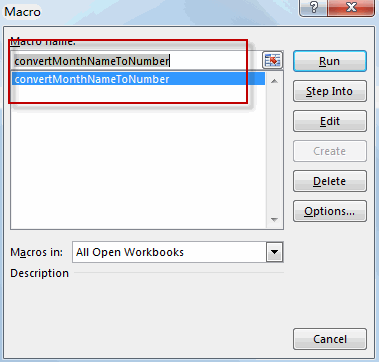#6 select cells that you want to convert, such as: B1:B12. Click Ok button.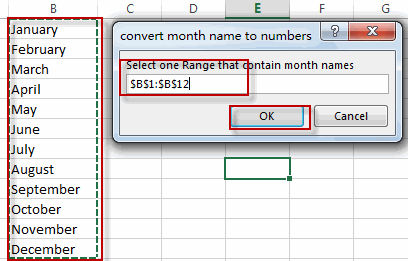#7 Let’s see the result.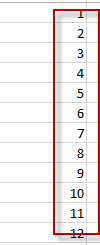## Convert Number to Month Name with Excel Formula

If you want to conert number to month name, you can use an formula based on the TEXT function, and the DATE function to achieve it. Like this:

`=TEXT(DATE(2018,B1,1),"mmmm")`

Type this formula into a blank cell, and then press enter key, then drag the AutoFill Handler over other cells.

Let’s see the result.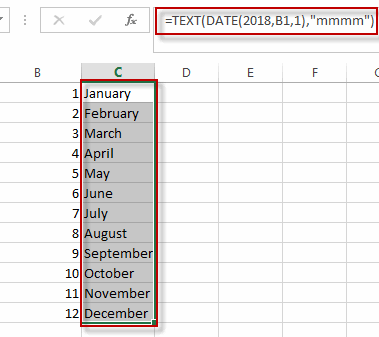### Related Functions

• Excel MONTH Function
The Excel MONTH function returns the month of a date represented by a serial number. And the month is an integer number from 1 to 12.The syntax of the MONTH function is as below:=MONTH (serial_number)…
• Excel DATEVALUE Function
The Excel DATEVALUE function returns the serial number of date. And it can be used to convert a date represented as text format into a serial number that recognizes as a date format.The syntax of the DATEVALUE function is as below:=DATEVALUE(date_text)…
• Excel DATE function
The Excel DATE function returns the serial number for a date.The syntax of the DATE function is as below:= DATE (year, month, day)…
• Excel Text function
The Excel TEXT function converts a numeric value into text string with a specified format. The TEXT function is a build-in function in Microsoft Excel and it is categorized as a Text Function. The syntax of the TEXT function is as below: = TEXT (value, Format code)…